### Home > CALC > Chapter 4 > Lesson 4.3.2 > Problem4-108

4-108.
1. Examine the following integrals. Consider the multiple tools available for evaluating integrals and use the best strategy for each. After evaluating the integral, write a short description of your method. Homework Help ✎

1.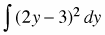2.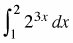3.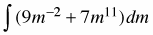4.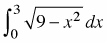5.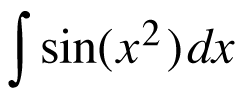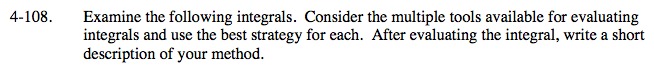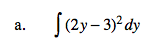Expand the expression first.

$\frac{4}{3}y^{3}-6y^{2}+9y+C$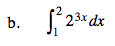Use a calculator.

≈26.93, using a calculator.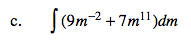How can you reverse the Power Rule?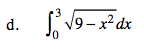Do NOT use a calculator.
What familiar geometric shape is made by the following equation?